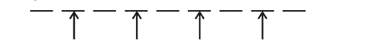# All possible numbers are formed using the digitsQuestion:

All possible numbers are formed using the digits $1,1,2,2$, $2,2,3,4,4$ taken all at a time. The number of such numbers in which the odd digits occupy even places is :

1. (1) 180

2. (2) 175

3. (3) 160

4. (4) 162

Correct Option: 1

Solution:

$\because$ There are total 9 digits and out of which only 3 digits are odd.$\therefore$ Number of ways to arrange odd digits first

$={ }^{4} C_{3} \cdot \frac{3 !}{2 !}$

Hence, total number of 9 digit numbers

$=\left({ }^{4} C_{3} \cdot \frac{3 !}{2 !}\right) \cdot \frac{6 !}{2 ! 4 !}=180$